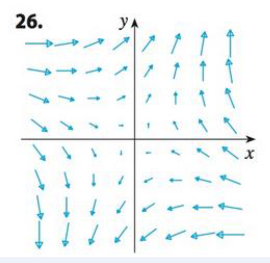Chapter 16.3, Problem 26E

Chapter
Section
Textbook Problem

Is the vector field shown in the figure conservative? Explain.To determine

To explain: Whether the vector field shown in the figure is conservative or not.

Explanation

Definition:

For a conservative vector field (F) , the line integral around any closed path C is zero. This is mathematically expressed as follows,

CFd<

Still sussing out bartleby?

Check out a sample textbook solution.

See a sample solution

The Solution to Your Study Problems

Bartleby provides explanations to thousands of textbook problems written by our experts, many with advanced degrees!

Get Started

Simplify the expressions in Exercises 97106. y5/4y3/4

Finite Mathematics and Applied Calculus (MindTap Course List)

Sometimes, Always, or Never: If c is a critical number, then f′(c) = 0.

Study Guide for Stewart's Single Variable Calculus: Early Transcendentals, 8th

The graph of is: a) b) c) d)

Study Guide for Stewart's Multivariable Calculus, 8th